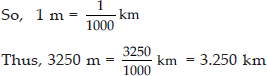# Motion and Measurement of Distances - NCERT - NCERT Questions

Q 1.

Give two examples each, of modes of transport used on land, water and air.

SOLUTION:

Modes of transport used on land:
(i) Car (ii) Bus
Modes of transport used on water:
(i) Ships (ii) Motorboats
Modes of transport used in air:
(i) Aeroplane (ii) Spacecraft

Q 2.

Fill in the blanks.
(i) One metre is _________ cm.
(ii) Five kilometre is ________ m.
(iii) Motion of a child on a swing is ________.
(iv) Motion of the needle of a sewing machine is ________.
(v) Motion of wheel of a bicycle is_______.

SOLUTION:

(i) One metre is 100 cm.
(ii) Five kilometre is 5000 m.
(iii) Motion of a child on a swing is periodic.
(iv) Motion of the needle of a sewing machine is periodic.
(v) Motion of wheel of a bicycle is circular.

Q 3.

Why can a pace or a footstep not be used as a standard unit of length?

SOLUTION:

A pace or a footstep cannot be used as a standard unit of length because everyone's body parts could be of slightly different sizes. So, measure of same length made by different people will be different.

Q 4.

Arrange the following lengths in their increasing magnitude.
1 metre, 1 centimetre, 1 kilometre, 1 millimetre.

SOLUTION:

1 millimetre < 1 centimetre < 1 metre < 1 kilometre.

Q 5.

The height of a person is 1.65 m. Express it into cm and mm.

SOLUTION:

As we know, 1 m = 100 cm
So, 1.65 m = 1.65 × 100 cm = 165 cm
Similarly, 1 cm = 10 mm
So, 165 cm = 165 × 10 mm = 1650 mm

Q 6.

The distance between Radha's home and her school is 3250 m. Express this distance into km.

SOLUTION:

Distance between Radha's home and her school = 3250 m
We know,
1 km = 1000 mQ 7.

While measuring the length of a knitting needle, the reading of the scale at one end is 3.0 cm and at the other end is 33.1 cm. What is the length of the needle?

SOLUTION:

Reading of the scale at one end = 3.0 cm
Reading of the scale at the other end = 33.1 cm
Length of the needle
= (33.1 – 3.0) cm = 30.1 cm

Q 8.

Write the similarities and differences between the motion of a bicycle and a ceiling fan that has been switched on.

SOLUTION:

Similarities between the motion of a bicycle and a ceiling fan:
(i) Blades of fan and tyres of bicycle, both are rotating.
(ii) Both are examples of periodic motion.
Difference between the motion of a bicycle and a ceiling fan:
Ceiling fan is undergoing rotational motion while bicycle is undergoing rectilinear as well as rotational motion.

Q 9.

Why could you not use an elastic measuring tape to measure distance? What would be some of the problems you would meet in telling someone about a distance you measured with an elastic tape?

SOLUTION:

Length of an elastic measuring tape can vary on stretching. So, it could not be used to measure distance. Some of the problems we would meet while measuring with an elastic tape are as follows:
(i) On measuring the length of an object twice, using an elastic tape, we may get different values of the same length.
(ii) Measurements will not be accurate.

Q 10.

Give two examples of periodic motion.

SOLUTION:

Examples of periodic motion:
(i) Pendulum clock
(ii) Guitar string